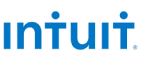New update is available. Click here to update.
Topics

# Append Nodes

Moderate0/80
Average time to solve is 38m## Problem statement

You have been given an integer Linked List.

After every 'M' nodes, you have to take the sum of the next 'N' nodes and append that sum to the linked list. Do this till the end of the Linked List.

Note:
``````You just need to return the head of the new linked list.

In case the linked list ends after adding K nodes, where K is any positive integer less than 'N'.Append the sum of those K nodes at the end of the linked list.
``````
Detailed explanation ( Input/output format, Notes, Images )
Constraints:
``````1 <= T <= 10
1 <= n <= 10^4
1 <= N <= 10^4
1 <= M <= 10^4
-10^5 <= VAL <= 10^5

Where 'n' denotes the length of the linked list and 'VAL' represents a node value of the linked list.

Time limit: 1 sec
``````
Sample Input 1:
``````2
1 2 3 4 5 6 7 8 9 10 11 12 -1
2 3
5 4 3 7 9 -1
4 3
``````
Sample Output 1:
``````1 2 3 4 5 9 6 7 8 9 10 19 11 12
5 4 3 7 9 16
``````
Explanation of the Sample Output 1:
``````In test case 1, for the linked list,  the value of 'M' is 3. So we skip the first 3 nodes(1->2->3). The value of 'N' is 2, hence we add the next 2 nodes(4->5). The sum is 9, so we add 9 after node 5. Now repeating the same process again, we skip the next 3 nodes(6->7->8). Now we add the next 2 nodes(9->10), for which the sum is 19. Hence we add 19 to the list after 10. Now while skipping the next 3 nodes we encounter the end of a linked list, hence the updated linked list is our answer.

In test case 2, for the linked list,  the value of 'M' is 3. So we skip the first 3 nodes(5->4->3). The value of 'N' is 4 so ideally we should add the next 4 nodes, but there are only two nodes remaining before we encounter the end of the linked list, hence we add those 2 nodes(7->9). The sum is 16, therefore we add 16 to the end of the linked list. Because we have encountered the end of the linked list, the updated linked list is our answer.
``````
Sample Input 2:
``````2
10 30 10 40 5 5 -1
2 3
1 -2 1 2 2 -4 2 4 -1
2 4
``````
Sample Output 2:
``````10 30 10 40 5 45 5
1 -2 1 2 2 -4 -2 2 4
``````
Explanation of the Sample Output 2:
``````In test case 1, for the linked list,  the value of 'M' is 3. So we skip the first 3 nodes(10->30->10). The value of 'N' is 2, hence we add the next 2 nodes(40->5). The sum is 45, so we add 45 after node 5. Now repeating the same process again, while skipping the next 3 nodes we encounter the end of a linked list, hence the updated linked list is our answer.

In test case 2, for the linked list,  the value of 'M' is 4. So we skip the first 4 nodes(1->-2->1->2). The value of 'N' is 2, hence we add the next 2 nodes(2->-4). The sum is -2, so we add -2 after node 6. Now repeating the same process again, while skipping the next 4 nodes we encounter the end of a linked list, hence the updated linked list is our answer.
``````Console